# Vertex AI Workbench：使用迁移学习和笔记本执行程序构建图片分类模型

## 学习内容

• 在笔记本中使用参数
• 通过 Vertex AI Workbench 界面配置和启动笔记本执行

## 2. Vertex AI 简介

Vertex AI 包含许多不同的产品，可支持端到端机器学习工作流。本实验将重点介绍 Vertex AI Workbench

Vertex AI Workbench 通过与数据服务（如 Dataproc、Dataflow、BigQuery 和 Dataplex）和 Vertex AI 深度集成，帮助用户快速构建基于笔记本的端到端工作流。它让数据科学家能够连接到 GCP 数据服务、分析数据集、试验不同的建模方法、将经过训练的模型部署到生产环境中，以及在整个模型生命周期内管理 MLOps。

## 第 2 步：启用 Vertex AI API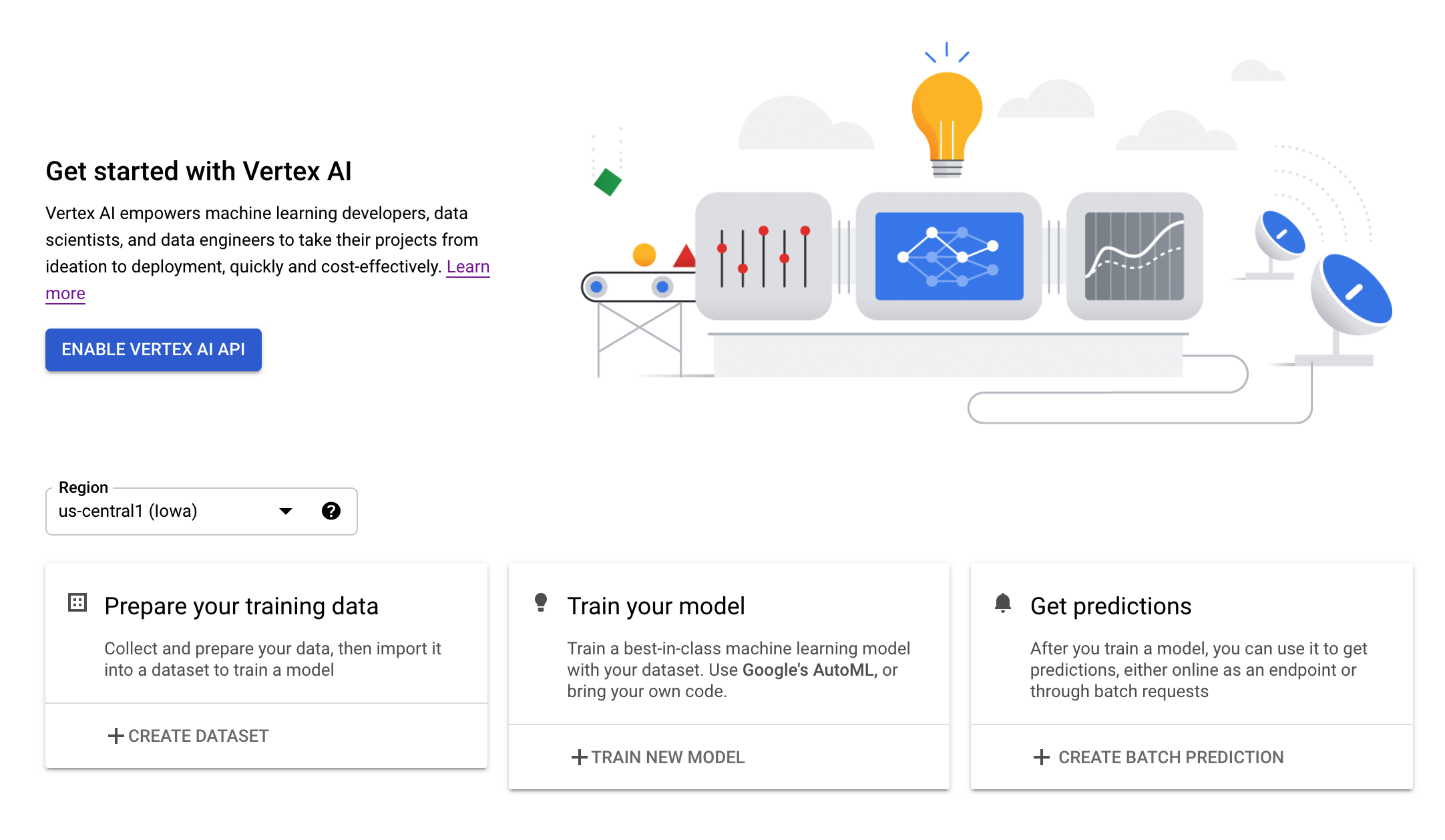## 第 3 步：创建 Vertex AI Workbench 实例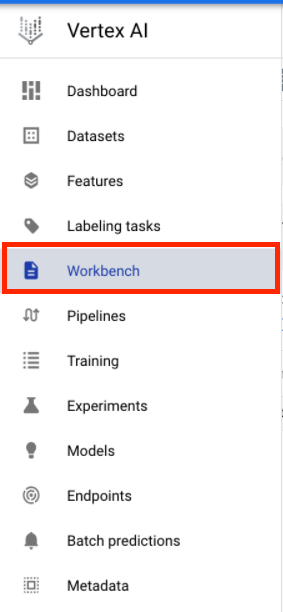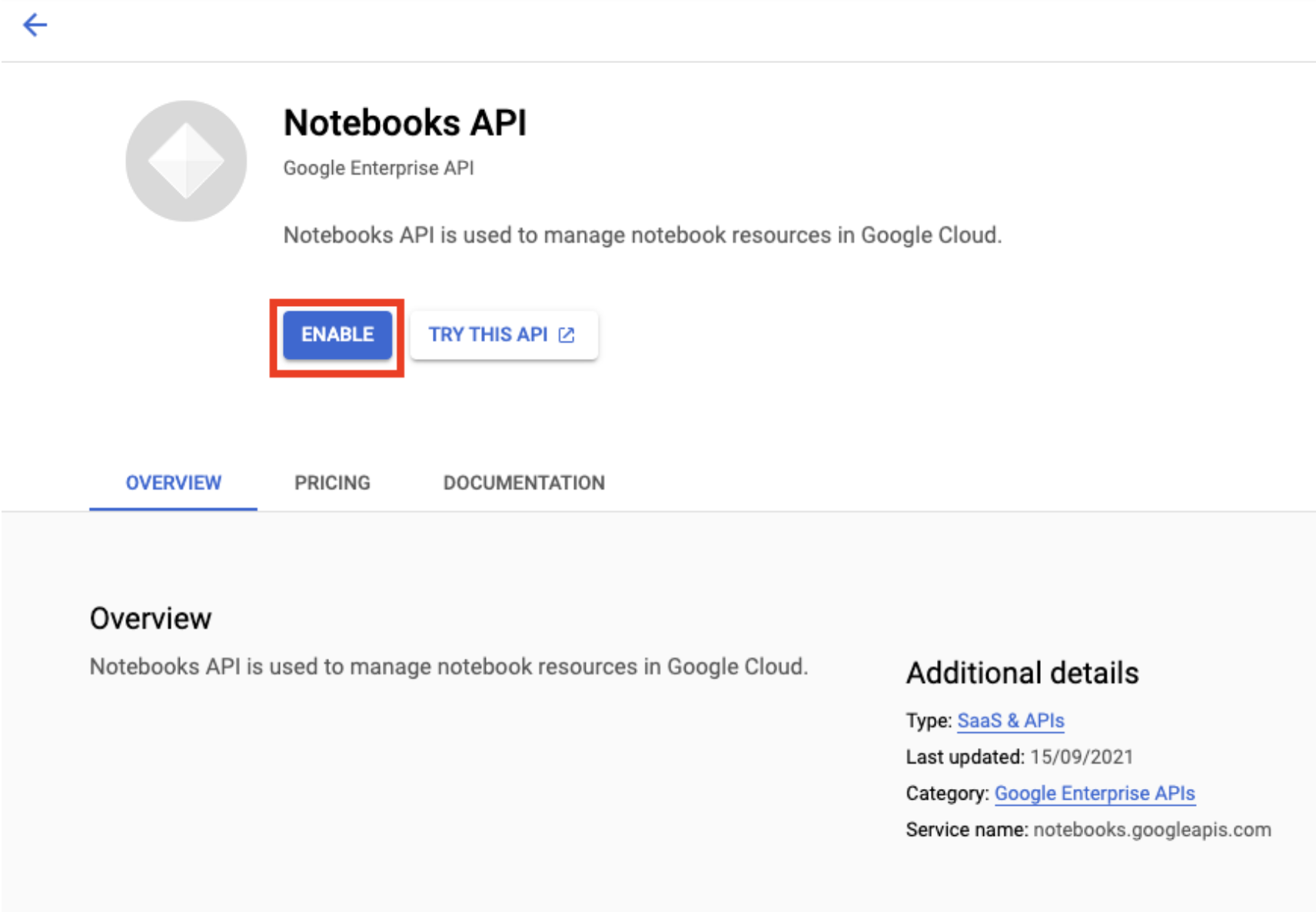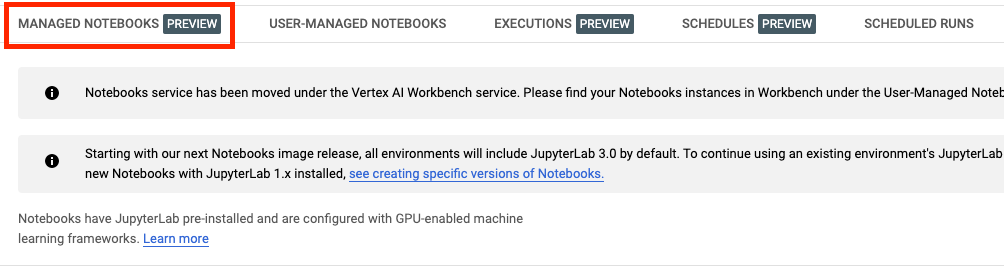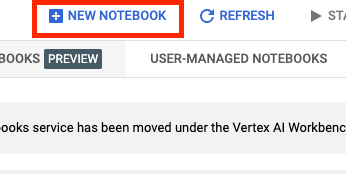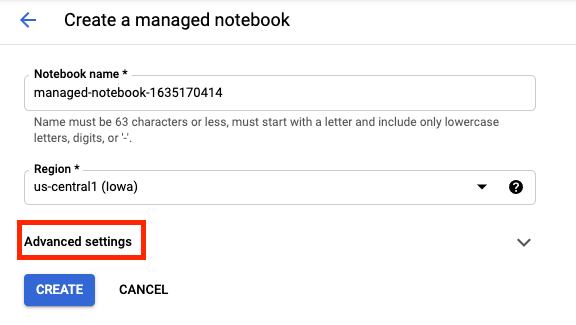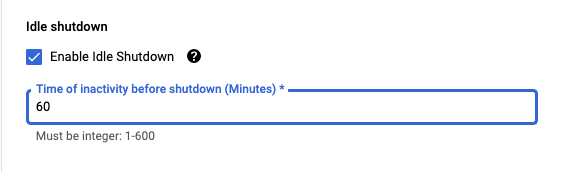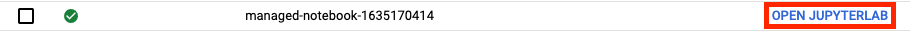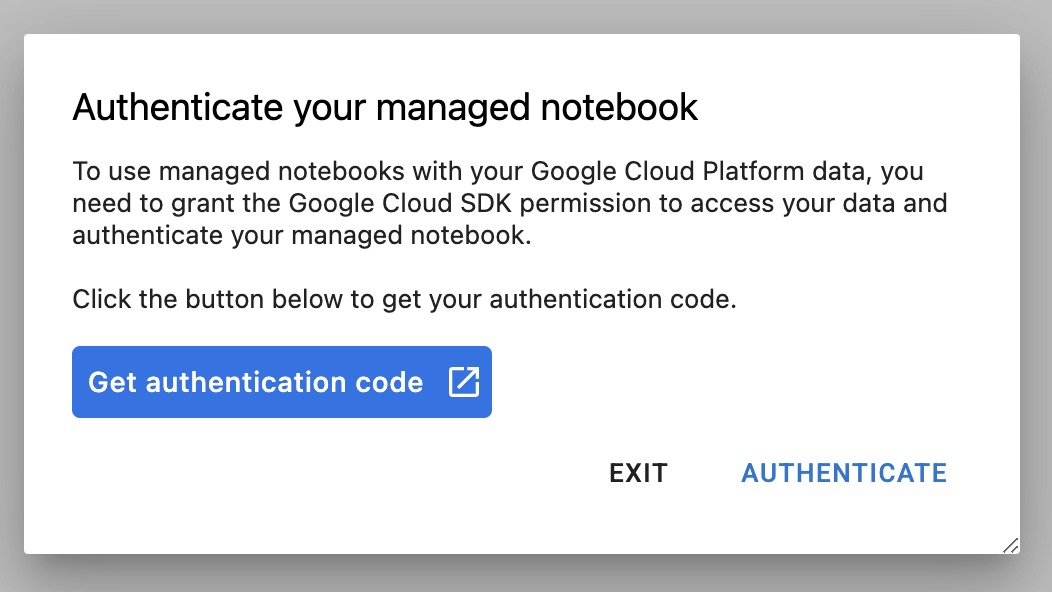Vertex AI Workbench 包含一个计算兼容性层，可让您通过一个笔记本实例启动适用于 TensorFlow、PySpark、R 等的内核。完成身份验证后，您将能够从启动器中选择要使用的笔记本类型。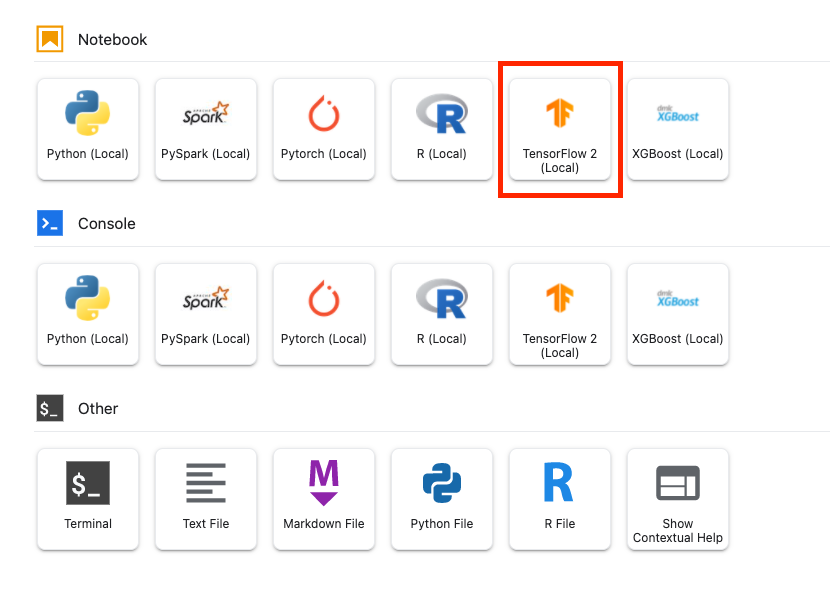## 5. 编写训练代码

DeepWeeds 数据集包含 17509 张图片，用于拍摄澳大利亚本土的八种不同杂草品种。在本部分中，您将编写代码，以预处理 DeepWeeds 数据集并使用从 TensorFlow Hub 下载的特征向量构建和训练图片分类模型。

## 第 1 步：下载和预处理数据集

``````import tensorflow as tf
import tensorflow_datasets as tfds
import tensorflow_hub as hub
``````

``````data, info = tfds.load(name='deep_weeds', as_supervised=True, with_info=True)
NUM_CLASSES = info.features['label'].num_classes
DATASET_SIZE = info.splits['train'].num_examples
``````

``````def preprocess_data(image, label):
image = tf.image.resize(image, (300,300))
return tf.cast(image, tf.float32) / 255., label
``````

DeepWeeds 数据集不包含训练/验证拆分。它只有一个训练数据集。在下面的代码中，您将利用其中 80% 的数据进行训练，其余 20% 用于验证。

``````# Create train/validation splits

# Shuffle dataset
dataset = data['train'].shuffle(1000)

train_split = 0.8
val_split = 0.2
train_size = int(train_split * DATASET_SIZE)
val_size = int(val_split * DATASET_SIZE)

train_data = dataset.take(train_size)
train_data  = train_data.map(preprocess_data)
train_data  = train_data.batch(64)

validation_data = dataset.skip(train_size)
validation_data  = validation_data.map(preprocess_data)
validation_data  = validation_data.batch(64)
``````

## 第 2 步：创建模型

``````feature_extractor_model = "inception_v3"
``````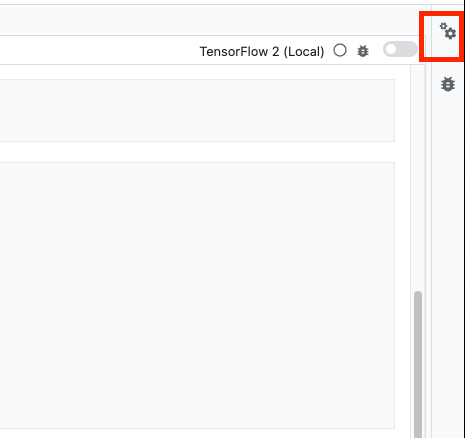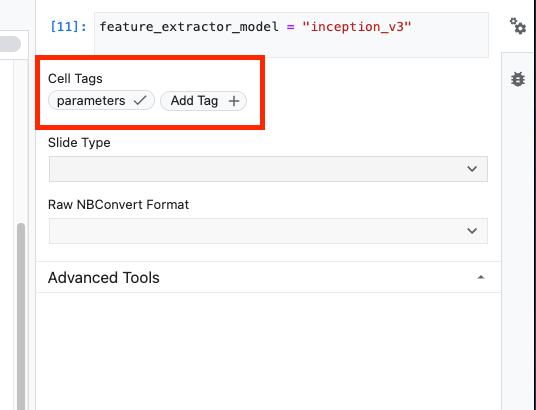``````tf_hub_uri = f"https://tfhub.dev/google/imagenet/{feature_extractor_model}/feature_vector/5"
``````

``````feature_extractor_layer = hub.KerasLayer(
tf_hub_uri,
trainable=False)
``````

``````model = tf.keras.Sequential([
feature_extractor_layer,
tf.keras.layers.Dense(units=NUM_CLASSES)
])
``````

``````model.compile(
loss=tf.keras.losses.SparseCategoricalCrossentropy(from_logits=True),
metrics=['acc'])

model.fit(train_data, validation_data=validation_data, epochs=20)
``````

## 6.执行笔记本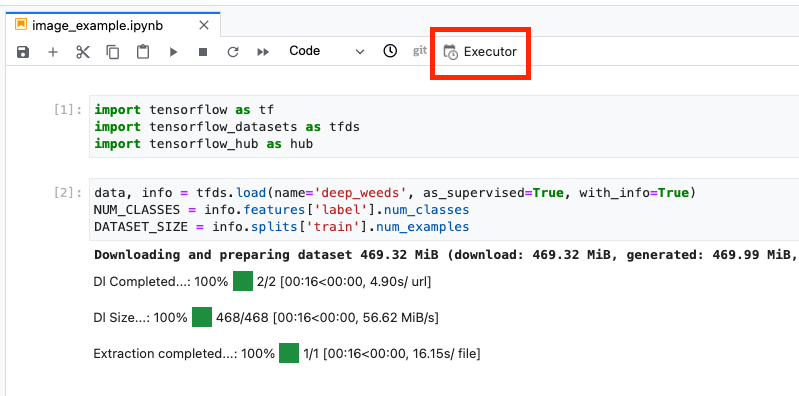## 第 1 步：配置训练作业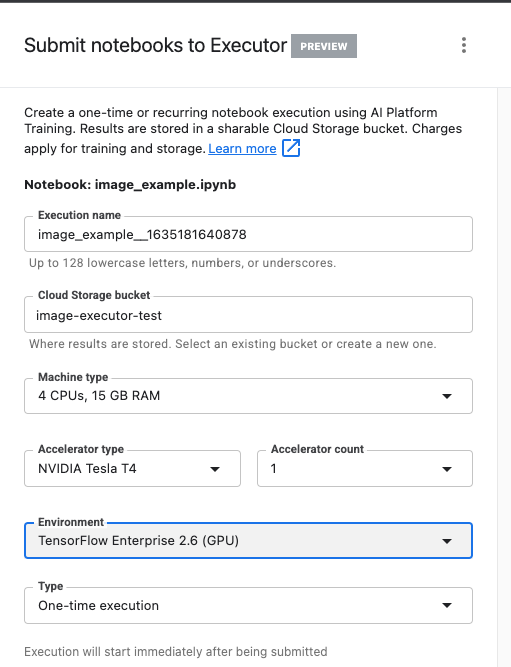## 第 2 步：配置参数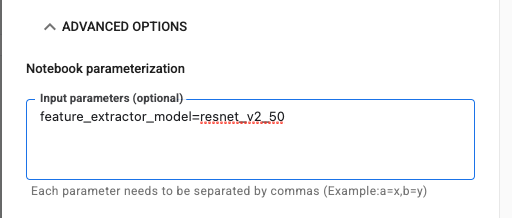## 第 3 步：检查结果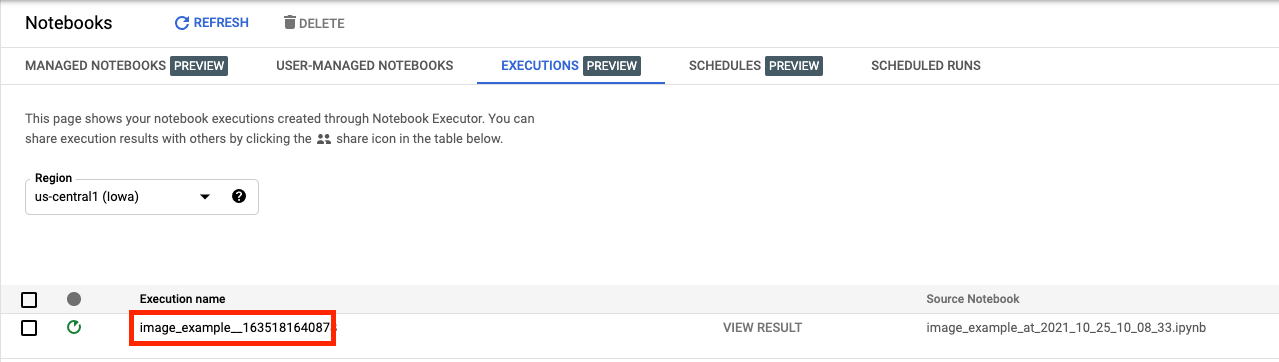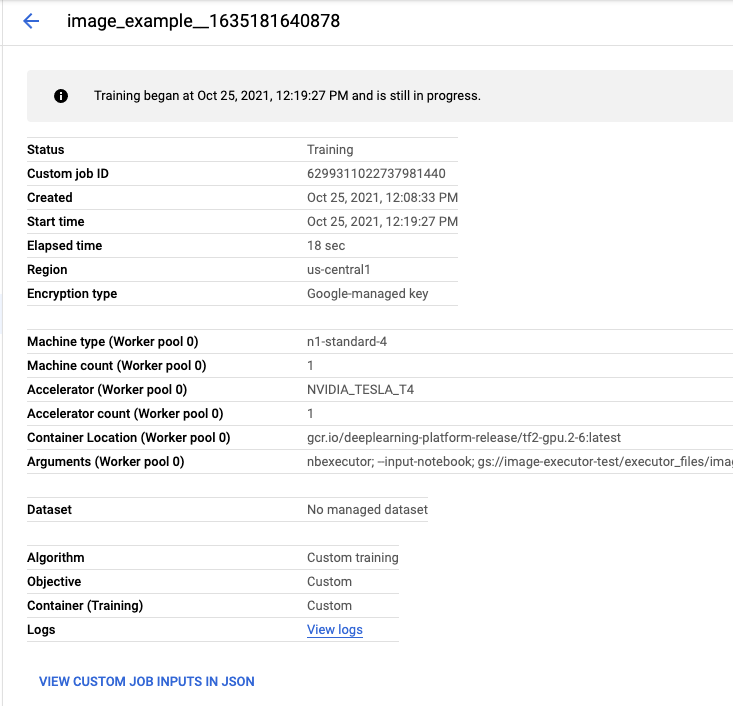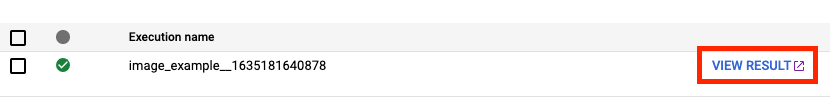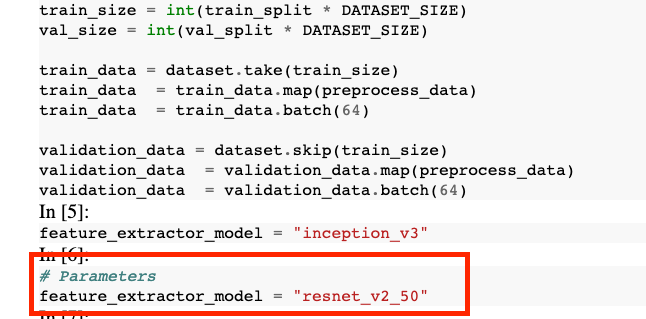🎉? 恭喜！🎉

• 在笔记本中使用参数
• 通过 Vertex AI Workbench 界面配置和启动笔记本执行

## 7. 清理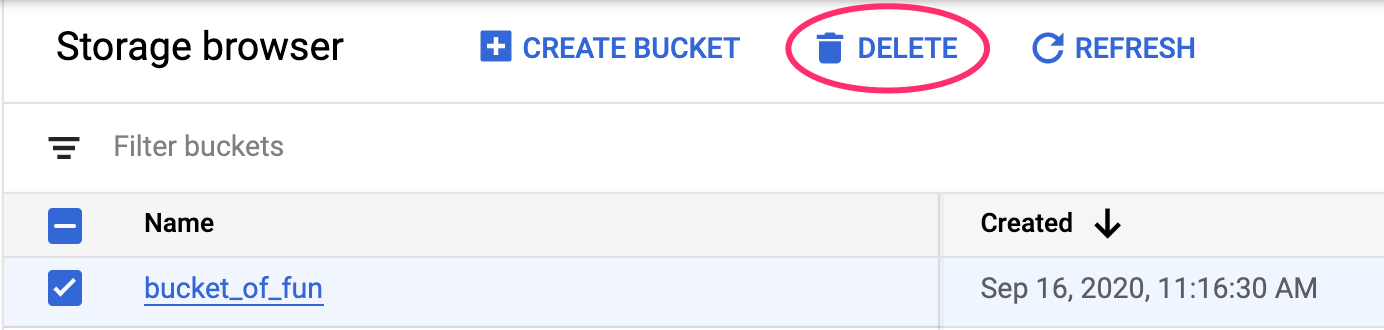[{ "type": "thumb-down", "id": "missingTheInformationINeed", "label":"没有我需要的信息" },{ "type": "thumb-down", "id": "tooComplicatedTooManySteps", "label":"太复杂/步骤太多" },{ "type": "thumb-down", "id": "outOfDate", "label":"内容需要更新" },{ "type": "thumb-down", "id": "translationIssue", "label":"翻译问题" },{ "type": "thumb-down", "id": "samplesCodeIssue", "label":"示例/代码问题" },{ "type": "thumb-down", "id": "otherDown", "label":"其他" }]
[{ "type": "thumb-up", "id": "easyToUnderstand", "label":"易于理解" },{ "type": "thumb-up", "id": "solvedMyProblem", "label":"解决了我的问题" },{ "type": "thumb-up", "id": "otherUp", "label":"其他" }]